何为站在巨人的肩膀上？

1. 明确的输入
2. 黑匣子（由输入到输出的映射关系）
3. 一个或多个明确的输出

准备数据集

ImageNet官网中的图片的种类和数量非常之多，为了研究问题，笔者选择5类物体（铃铛，狗狗，高尔夫球，校车和iPod），每一类物体大约1000多个。所以，我们需要将所有下载的图片处理成4维张量，其格式为（图片数量，长，宽，3），其中由于图片是彩色的具有RGB值，自然张量最后一位是3，即通道数。如下是处理大量图片的部分代码，笔者代码并非最优，只是解决笔者需要的图片处理问题。

import cv2
import numpy as np

from skimage import io

image = cv2.resize(image, (60, 60))
image = image * 1.0 / 255
image = np.array(image)
if image.shape != (60, 60, 3):
file_object.write(trainFile + '\n')
continue
image = image.reshape(1, 60, 60, 3)
feature = np.concatenate((feature, image), axis=0)
import numpy as np

trainfile = open("train_label.txt","r")
label = []
for i in range(len(line)):
label.append(int(line[i]))
trainfile.close()
Y_train = np.array(label)
Y_train = np.eye(5)[Y_train]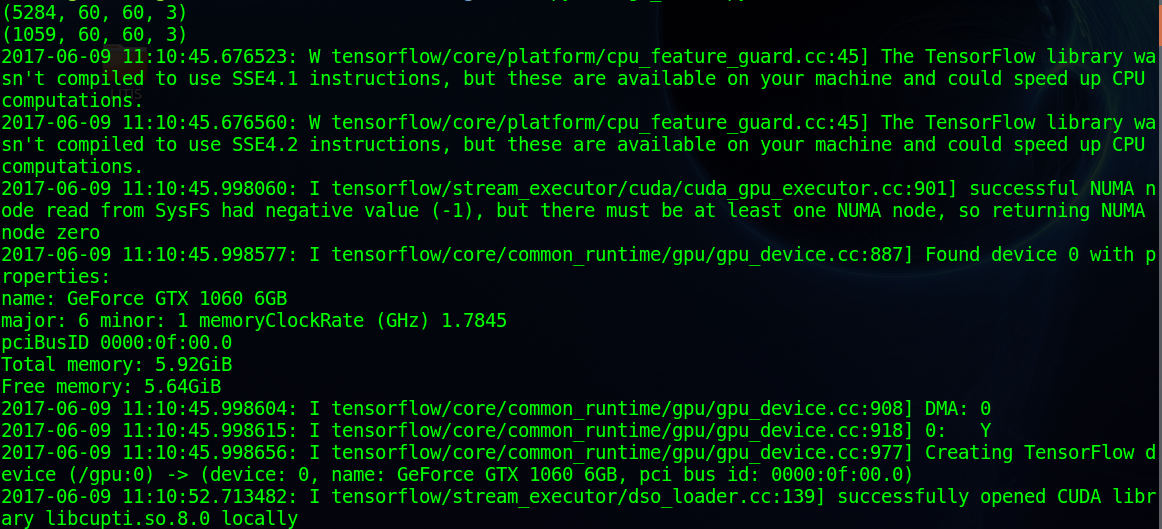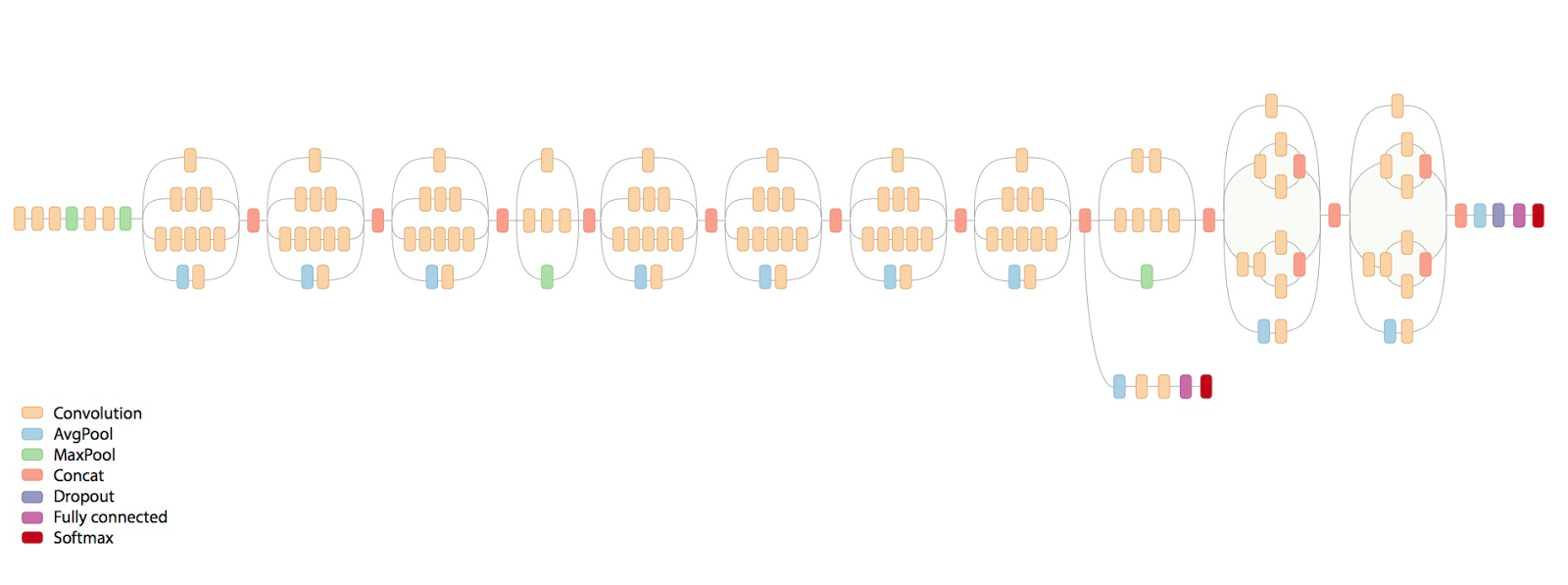1. 1×1的卷积操作
2. 1×1的卷积操作后再经过3×3的卷积操作
3. 1×1的卷积操作后再经过5×5的卷积操作
4. 3×3的最大池化操作后再经过1×1的卷积操作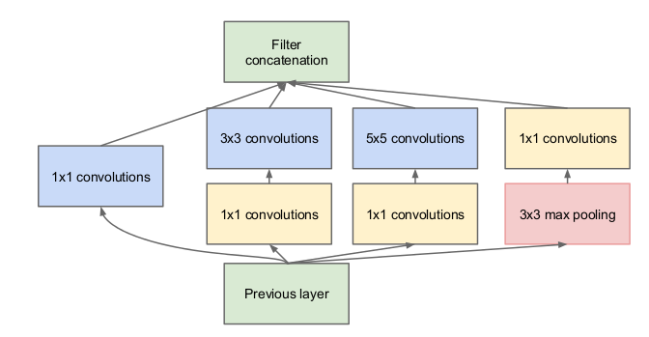#Layer Inception
with tf.name_scope('layer2-inception'):
with tf.variable_scope('conv2_1'):
W_conv2_1x1_1 = tf.Variable(tf.truncated_normal([1, 1, kernels1, 32], dtype=tf.float32,
stddev=1e-1), name='weights')
variable_summaries(W_conv2_1x1_1, 'W_conv2_1x1_1')
b_conv2_1x1_1 = tf.Variable(tf.constant(0.0, shape=, dtype=tf.float32),
trainable=True, name='biases')
variable_summaries(b_conv2_1x1_1, 'b_conv2_1x1_1')
conv2_1x1_1 = tf.nn.conv2d(pool1, W_conv2_1x1_1, [1, 1, 1, 1], padding='SAME')
conv2_1x1_1_sum = tf.nn.bias_add(conv2_1x1_1, b_conv2_1x1_1)
conv2_1x1_1_relu = tf.nn.relu(conv2_1x1_1_sum)
with tf.variable_scope('conv2_2'):
with tf.variable_scope('conv2_2_1x1'):
W_conv2_1x1_2 = tf.Variable(tf.truncated_normal([1, 1, kernels1, 32],
dtype=tf.float32,stddev=1e-1), name='weights')
variable_summaries(W_conv2_1x1_2, 'W_conv2_1x1_2')
b_conv2_1x1_2 = tf.Variable(tf.constant(0.0, shape=, dtype=tf.float32),
trainable=True, name='biases')
variable_summaries(b_conv2_1x1_2, 'b_conv2_1x1_2')
conv2_1x1_2 = tf.nn.conv2d(pool1, W_conv2_1x1_2, [1, 1, 1, 1], padding='SAME')
conv2_1x1_2_sum = tf.nn.bias_add(conv2_1x1_2, b_conv2_1x1_2)
conv2_1x1_2_relu = tf.nn.relu(conv2_1x1_2_sum)
with tf.variable_scope('conv2_2_3x3'):
W_conv2_3x3_2 = tf.Variable(tf.truncated_normal([3, 3, 32, 32], dtype=tf.float32,
stddev=1e-1), name='weights')
variable_summaries(W_conv2_3x3_2, 'W_conv2_3x3_2')
b_conv2_3x3_2 = tf.Variable(tf.constant(0.0, shape=, dtype=tf.float32),
trainable=True, name='biases')
variable_summaries(b_conv2_3x3_2, 'b_conv2_3x3_2')
conv2_3x3_2 = tf.nn.conv2d(conv2_1x1_2_relu, W_conv2_3x3_2, [1, 1, 1, 1], padding='SAME')
conv2_3x3_2_sum = tf.nn.bias_add(conv2_3x3_2, b_conv2_3x3_2)
conv2_3x3_2_relu = tf.nn.relu(conv2_3x3_2_sum)

with tf.variable_scope('merge'):
inception = tf.nn.relu(tf.concat(3, [conv2_1x1_1_relu,
conv2_3x3_2_relu,
conv2_5x5_3_relu,
conv_1x1_4_relu]))

Inception层中我们会得到四个模块最后的输出：

• conv2_1×1_1_relu
• conv2_3×3_2_relu
• conv2_5×5_2_relu
• conv_1×1_4_relu

sess = tf.InteractiveSession()
merged = tf.summary.merge_all()
saver = tf.train.Saver()
train_writer = tf.summary.FileWriter('train/', sess.graph)
test_writer = tf.summary.FileWriter('test/', sess.graph)
run_options = tf.RunOptions(trace_level = tf.RunOptions.FULL_TRACE)
tf.global_variables_initializer().run()

for i in range(60000):

if i % 10 == 0:    # Record summaries and test-set accuracy
summary, acc = sess.run([merged, accuracy], feed_dict = feed_dict(False),
print("step %d, training accuracy %g" %(i, acc))
else:    # Record train set summaries, and train
if i % 100 == 99:    # Record execution stats
summary, _ = sess.run([merged, train_step], feed_dict = feed_dict(True),
else:
summary, _ = sess.run([merged, train_step], feed_dict = feed_dict(True))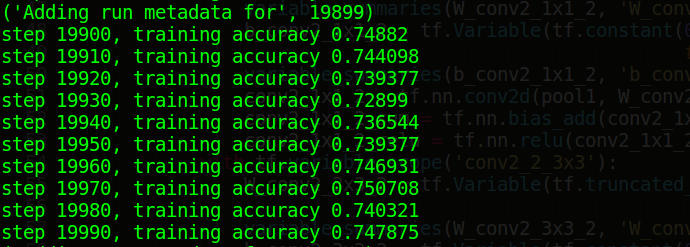def feed_dict(train):
if train:
batch_xs, batch_ys = next_batch(X_train, Y_train, batch_size)
k = 0.5
else:
batch_xs, batch_ys = X_test, Y_test
k = 1.0
return {x: batch_xs, y_: batch_ys, drop_rate: k}

​
def next_batch(feature, label, _batch_size):
num_examples = len(feature)
index_in_epoch = 0

start = index_in_epoch
index_in_epoch += _batch_size
if index_in_epoch < num_examples:
perm = np.arange(num_examples)
np.random.shuffle(perm)
feature = feature[perm]
label = label[perm]
start = 0
index_in_epoch = _batch_size
assert _batch_size <= num_examples
end = _batch_size
return feature[start:end], label[start:end]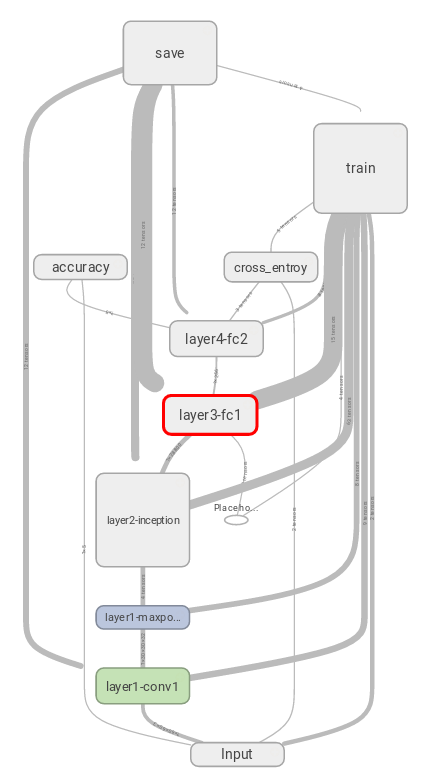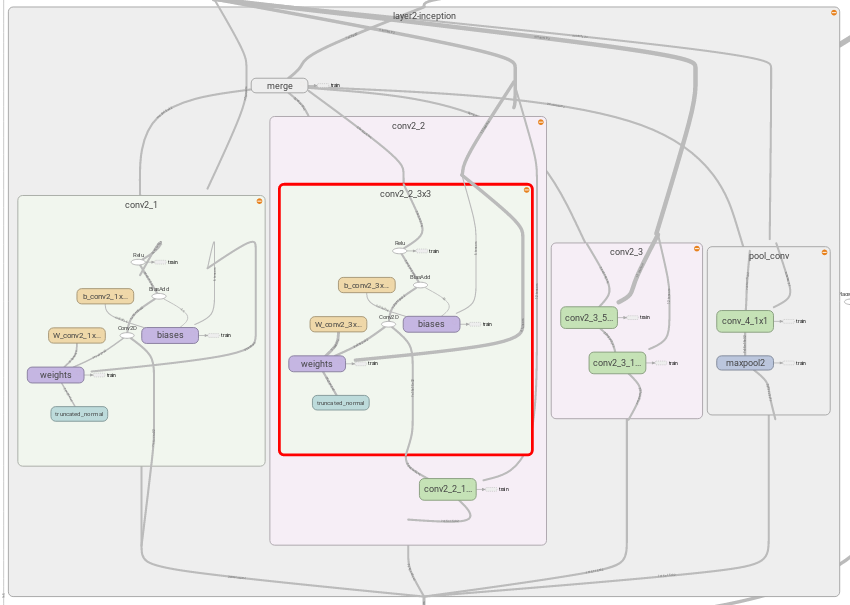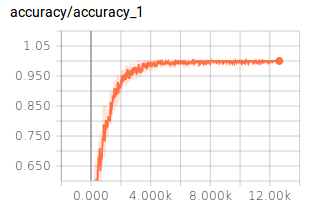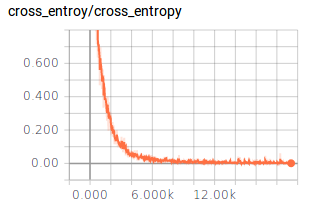还想说点什么...

Negative！

Negative！

（虽然GTX1060 6G已经满给力了，毕竟人家是用至少4个Titan来训练的）

nextowang@stu.xidian.edu.cnnextowang

2017/08/23
0
0

manofmountain
2018/08/11
0
0

2016/09/26
7.5K
0

navychang
2016/04/15
4
0
GPU云服务器深度学习性能模型初探

1 背景 　　得益于GPU强大的计算能力，深度学习近年来在图像处理、语音识别、自然语言处理等领域取得了重大突破，GPU服务器几乎成了深度学习加速的标配。 　　阿里云GPU云服务器在公有云上提...

2018/04/23
0
0

sync.Mutex 互斥锁

3分钟前
1
0

6分钟前
2
0

Sentinel 承接了阿里巴巴近 10 年的双十一大促流量的核心场景 本文介绍阿里开源限流熔断方案Sentinel功能、原理、架构、快速入门以及相关框架比较 基本介绍 1 名词解释 服务限流 ：当系统资源...

8分钟前
1
0

svn co URL --username xxx-- password yyy ./

31分钟前
2
0

shzwork
32分钟前
2
0